Computational & Technology Resources
an online resource for computational,
engineering & technology publications
Civil-Comp Proceedings
ISSN 1759-3433
CCP: 73
PROCEEDINGS OF THE EIGHTH INTERNATIONAL CONFERENCE ON CIVIL AND STRUCTURAL ENGINEERING COMPUTING
Edited by: B.H.V. Topping
Paper 88

Sensitivity of Inverse Boundary Element Techniques to Errors in Photoelastic Measurements

P. Wang, A.A. Becker, I.A. Jones and T.H. Hyde

School of Mechanical, Materials, Manufacturing Engineering and Management, University of Nottingham, United Kingdom

Full Bibliographic Reference for this paper
P. Wang, A.A. Becker, I.A. Jones, T.H. Hyde, "Sensitivity of Inverse Boundary Element Techniques to Errors in Photoelastic Measurements", in B.H.V. Topping, (Editor), "Proceedings of the Eighth International Conference on Civil and Structural Engineering Computing", Civil-Comp Press, Stirlingshire, UK, Paper 88, 2001. doi:10.4203/ccp.73.88
Keywords: boundary element method, photoelasticity, stress separation, inverse problem.

Summary
The application of computational mechanics techniques to inverse problems has been receiving increasing attention in various branches of science and engineering. In solid mechanics it may provide non-destructive evaluation tools which can be used to identify unknown variables at the surface, such as the stress distribution in a contact region between contacting bodies. Photoelastic readings provide information regarding the differences of the principal stresses and their orientations at interior points. However, it is often difficult to separate the individual Cartesian stress components from the photoelastic data The BE technique is an ideal companion to photoelastic analysis since, unlike the finite element (FE) method, the interior solutions can be represented by unconnected points rather than discretised elements.

This paper presents an inverse boundary element (BE) technique to reconstruct the boundary conditions in the unknown regions using photoelastic measurements taken from the photoelastic isochromatic fringe order and the isoclinic angle at interior points. The separate individual Cartesian stress components are then obtained using the forward BE method The BE approach is based on two integral equations: a surface (boundary) integral equation relating displacements and tractions on the surface, and an interior integral equation relating the stresses at the interior points to the surface displacements and surface tractions. The surface (boundary) integral equation is as follows: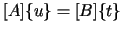(88.1)

where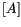and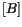are matrices that contain the numerical integrations involving the surface kernels. After applying the boundary conditions of the problem, the relevant elements of the matricesandare swapped such that all the unknowns end up on the left hand side of the equation and the known variables are on the right hand side, as follows: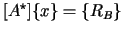(88.2)

where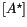is the solution matrix,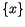contains all unknown variables (displacements and tractions), and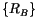contains all prescribed values of displacements and tractions. After solving the equations, all tractions and displacements on the boundary are known. The interior integral equation is as follows: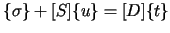(88.3)

where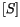and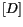are matrices that contain the numerical integrations involving the interior kernels. Therefore, in the forward BE approach, the boundary displacements and tractions are computed first, and then subsequently used to obtain the interior variables. The inverse method, as the name implies, uses the interior stress values to compute the boundary values. By applying the prescribed boundary conditions and swapping the relevant elements of the matricesand, Equation 88.3 becomes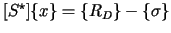(88.4)

Previous research has shown that inverse BE methods relying on the interior kernels only cannot be used to obtain the solution, and that it is necessary to use both interior integral equation 88.4 and surface integral equation 88.2. In the unknown region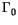, both the displacements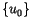and tractions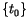are unknown. If one set of them is obtained first the remaining unknowns can be solved as the forward BE procedure. The one which is to be found first will appear on the right-hand side in equations 88.2 and 88.4 respectively, as follows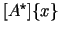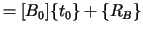(88.5)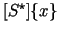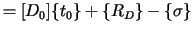(88.6)

Subscript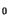denotes the related partitions belonging to the unknown regionof that matrix or vector. Combining equations 88.5 and 88.6 and eliminatingfrom them, an equation within regionas the only unknowns, can be derived as follows: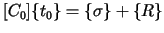(88.7)

where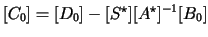(88.8)

and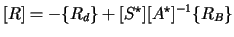(88.9)

The Singular Value Decomposition (SVD) method is used to solve equation 88.7. It is clear that onceis computed from equation 88.7, the remaining unknowns can be solved straightforwardly using the forward BE equation 88.5. The present method to deal with photoelastic stress analysis by employing inverse BEM described above is to create the interior equations at a number of chosen points in equation 88.6 by using the difference of Cartesian direct stresses and the magnitude of the Cartesian shear stress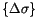. Accordingly, the coefficients in the matricesandare replaced by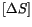and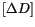respectively. This paper also investigates the sensitivity of the inverse BE technique to errors of photoelastic data at the interior points, discusses how to choose the number and locations of the interior points and how to improve the numerical algorithm. The study indicates that the number of measurement points and the location of these points are important in constructing an accurate system matrix and dealing with the measurement errors. A criterion for locating the interior measurement points is given. Examples show good agreement with other solutions even if up to 5% 'noise' is added to the photoelastic measurement data.

purchase the full-text of this paper (price £20)